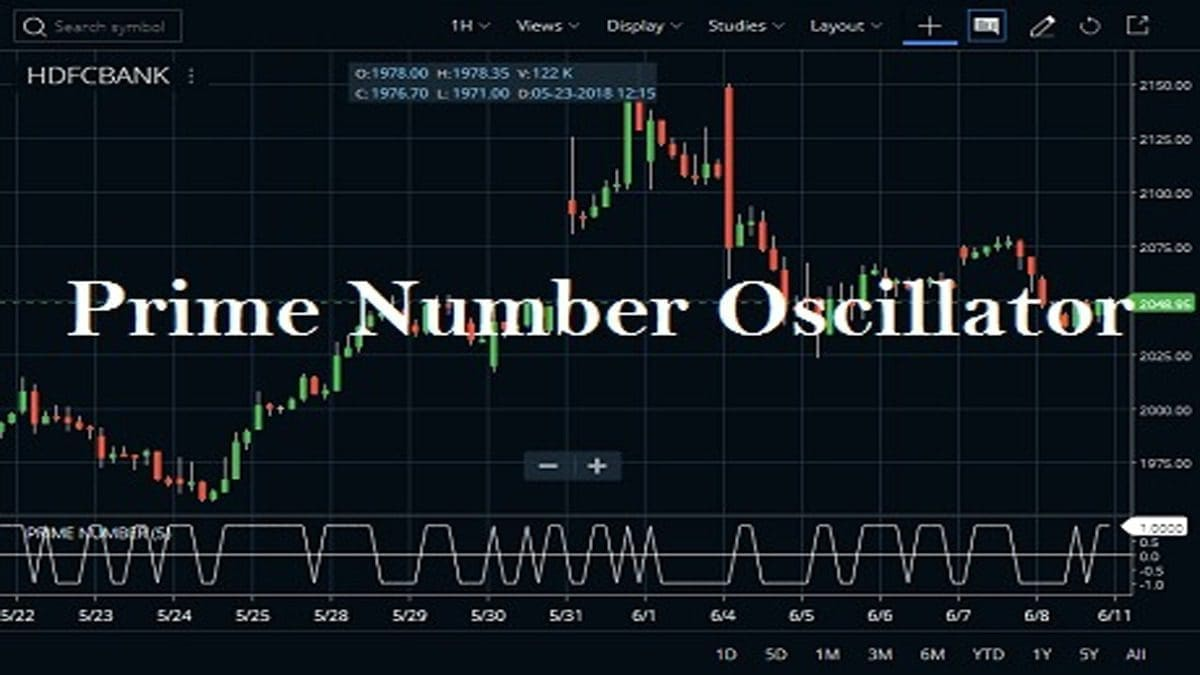# Prime Number Oscillator Indicator Strategy, FormulaThe prime number oscillator indicator indicates the nearest prime number, be it at the top or the bottom of the series, and outlines the difference between that prime number and the respective series. Like its band version, the oscillator was also developed by Modulus Financial Engineering Inc. Determining market trends has become a science even though a high number of people still believe it’s a gambling game. In order to understand and predict market movements, technicians and mathematicians along with brokers and investors developed several indicators.

If traders want to know more about the Prime Number Oscillator indicator, they can find it in the STUDIES section of Zerodha Kite. They can also use the Kite Mobile App to find out the indicator. The Tolerance Percentage is 5 and we can also set the color. Check the image below to understand how to attach the prime number oscillator indicator in HDFC Bank share price chart. You can attach this indicator on to any charts likes daily, weekly, monthly or intraday.

## How to use the Prime Number Oscillator Indicator :

The traders call prime an integer positive number that has no positive integer divisors other than 1 and itself, so it is a positive integer (greater than 1) that has exactly one divisor other than 1. As an example, 17 can be divided by 1 and 17 – it’s prime whereas 18 can be divided by 1, 2, 3, 6, 9 and 18 – it’s not prime. These numbers that appear in a specific order are said to create a sequence. As a sequence contains elements (terms), but unlike a set, the terms of a sequence follow a specific rule, are situated in a certain order. Mathematically, the Fibonacci numbers create the following integer sequence is : (0,1,1,2,3,5,8,13,21….). Traders use this sequence to determine future market movements. By using various methods the Fibonacci sequence is applied and Fibonacci Box consists of these methods. The box contains the Fibonacci extensions, retracements, circles, fan and time methods. By using the numbers in this mentioned sequence traders manage to time specific turns in the market movements.

The most popular way to use prime numbers in trading is through the prime number oscillator. It is a highly useful technical indicator in identifying changes in the market. The difference between the nearest prime number and the evolution of the prices series are it is easy to see when it is better to buy or sell. The indicator oscillates between +1 and -1. Whenever this indicator is constant and at a high value in the positive chart, the best choice is to sell. Otherwise, when the indicator is low for two consecutive periods and is situated in the negative range, an acquisition is recommended.

Prime Number Oscillator Indicator Strategy, Formula

This site uses Akismet to reduce spam. Learn how your comment data is processed.

Scroll to top